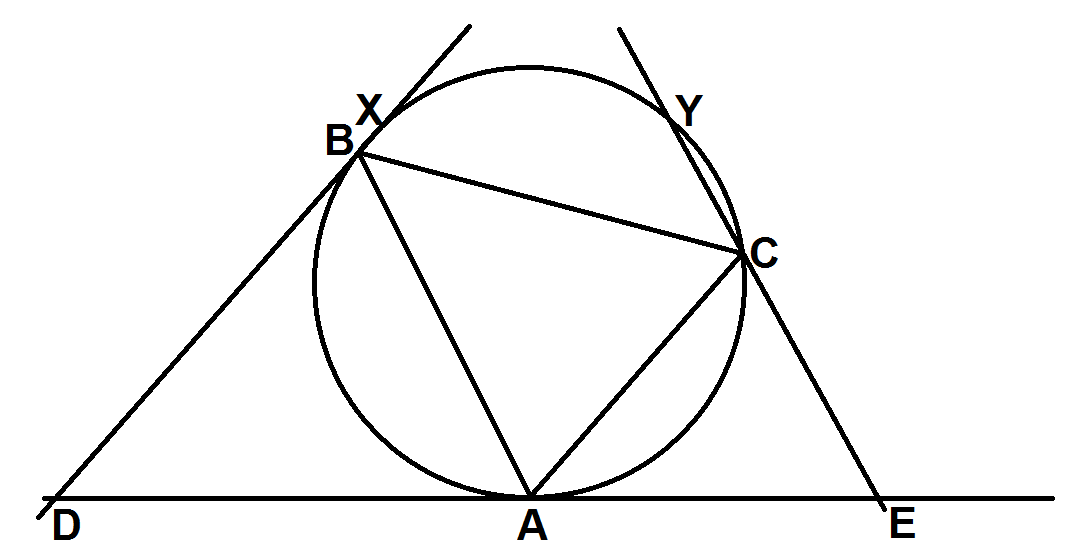# Tangents and Triangles

Geometry Level 4The tangent at $A$ of the circumcircle of the triangle $ABC$ is drawn. The points $D$ and $E$ are constructed on it such that $BD$ is parallel to $CA$ and that $CE$ is parallel to $BA$. The lines $BD$ and $CE$ intersect the circle $ABC$ again at $X$ and $Y$ respectively.

Given that $BC = 10$, find $AX + AY$.

×